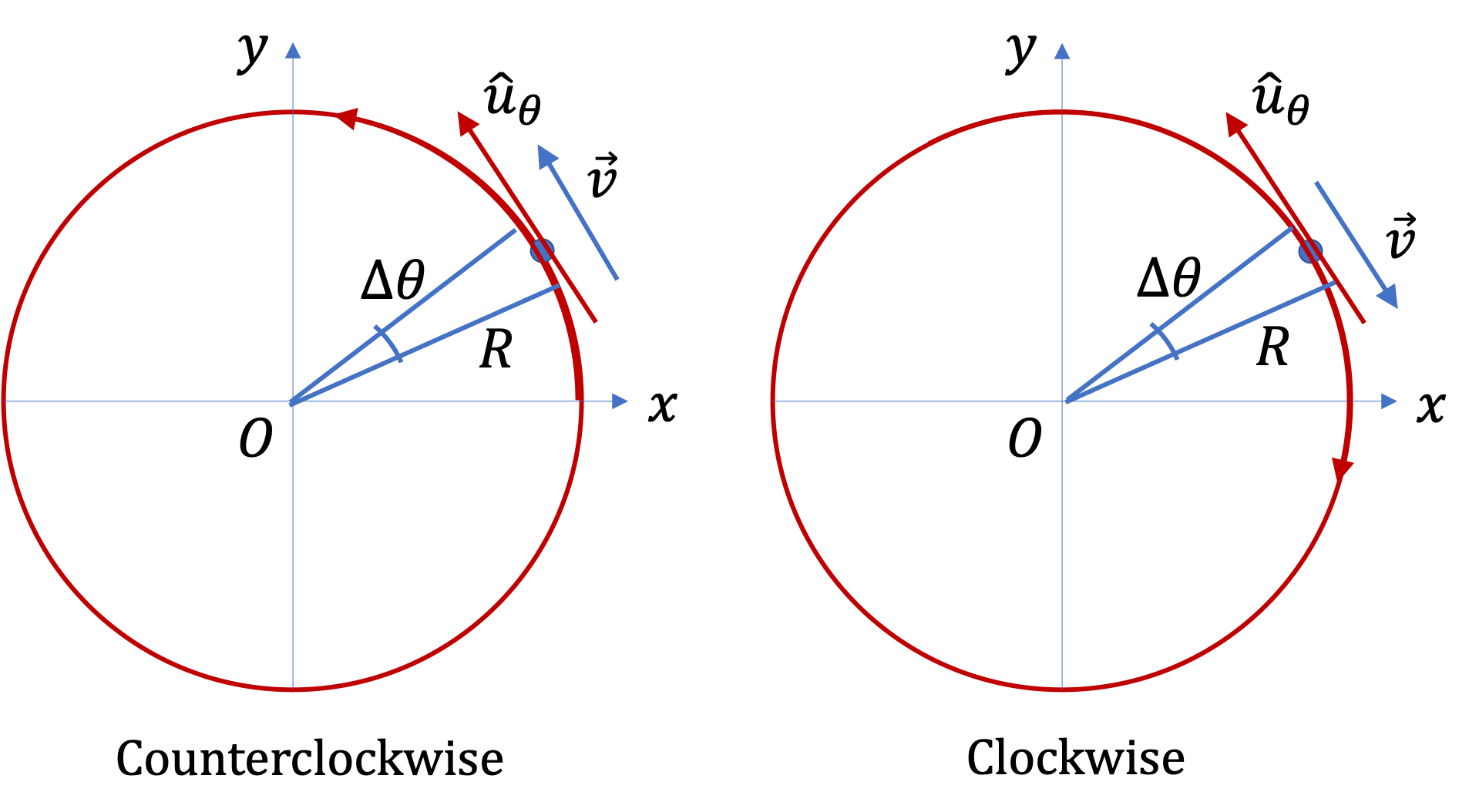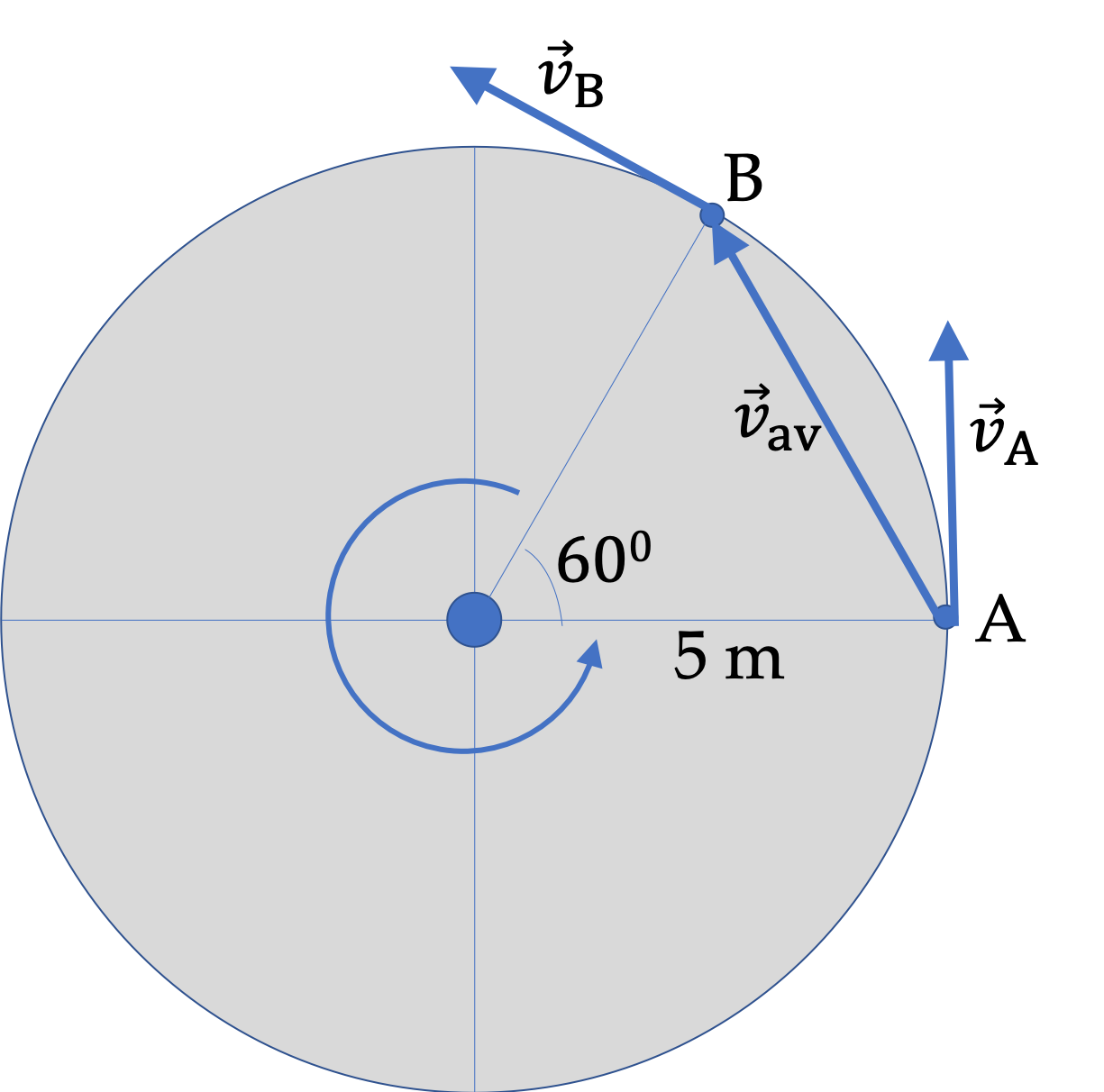## Section5.2Velocity of a Circular Motion

You know that average velocity is displacement divided by interval.

\begin{equation*} \vec v_\text{av} = \dfrac{\Delta \vec r}{\Delta t}. \end{equation*}

For infinitesimal intervals, this formula defines instantaneous velocity. We found in previous section that for small intervals in a circular motion, the displacment is

\begin{equation*} \Delta \vec r = R \Delta \theta\, \hat u_\theta, \end{equation*}

where direction is given by unit vector $\hat u_\theta\text{,}$ the direction of tangent to the circle. Therefore, average velocity for small time intervals will be

\begin{equation*} \vec v_\text{av} = R \dfrac{\Delta \theta}{\Delta t}\; \hat u_\theta. \end{equation*}

The quantity $\dfrac{\Delta \theta}{\Delta t}$ is called average angular “velocity” although it is not a vector. It is denoted by the Greek letter omega, $\omega_\text{av}\text{.}$

\begin{equation*} \omega_\text{av} = \dfrac{\Delta \theta}{\Delta t}. \end{equation*}

By convention, $\Delta \theta \gt 0$ for motion in the counterclockwise sense. Velocity $\vec v$ is in the tangetial direction in the unit vector $\hat u_\theta$ direction for motion in counterclockwise sense. On the other hand, when the motion is in the clockwise sense, $\Delta \theta \lt 0\text{,}$ and while velocity is still tangetial to circle, but it will be in opposite direction to the unit vector $\hat u_\theta$ direction.Figure 5.2.2. Velocity for counterclockwise sense is in the direction of the tangential unit vector $\hat u_\theta$ and in the opposite direction for clockwise sense of motion.

This can be used to replace the derivative in instantaneous velocity giving us a compact formula.

\begin{equation*} \vec v = R \omega\, \hat u_\theta. \end{equation*}

Note the factor $R \omega$ in velocity is analogous to the arc length $R\theta\text{.}$ Thus, instantaneous velocity has magnitude $R|\omega|\text{,}$ which is instantaneous speed $v\text{,}$ and direction in the direction of the tangent to the circle.

\begin{equation*} v = R|\omega|, \end{equation*}

where I have placed absolute sign to denote angular speed, which should be positive, regardles sof the clockwise or coungercloskwise sense of motion.

###### Remark5.2.3.

(Calculus) When we take infinitesimal interval limit, we get velocity at instant $t$ as derivative

\begin{equation*} \vec v = R \frac{d \theta}{d t}\, \hat u_\theta. \end{equation*}

The instantaneous rate of change of subtended angle is called instantaneous angular velocity, which is denoted by $\omega\text{.}$

\begin{equation*} \omega = \frac{d \theta}{d t}. \end{equation*}

A car is rounding a circular bend of radius $50\text{ m}$ with speed $20\text{ m/s}\text{.}$ What is the angular speed of the car?

Hint

$v = R|\omega|$

$0.4\text{ rad/s}\text{.}$

Solution

We use $v = R|\omega|$ to find angular speed.

\begin{align*} |\omega| \amp = \dfrac{v}{R}\\ \amp = \dfrac{20\text{ m/s}}{50\text{ m}} = 0.4\text{ rad/s}. \end{align*}

Note that I put $\text{rad}$ in the unit for $|\omega|$ since the displacement it refers to is the angle subtended expressed in radians.

A car is moving in a circular path of radius $40\text{ m}$ with increasing speed. Its angular position is given by $\theta = 0.05\, t^2$ in radians when $t$ is in seconds. (a) What is angular speed at $t=3\text{ s}\text{?}$ (b) (a) What is speed at $t=3\text{ s}\text{?}$

Hint

(a) Use $\omega = d\theta/dt\text{,}$ (b) Use $v = R|\omega|\text{.}$

(a) $0.3\text{ rad/s}\text{,}$ (b) $12\text{ m/s}\text{.}$

Solution

(a) Angular speed will be absolute value of

\begin{equation*} \omega = \dfrac{d\theta}{dt} = 0.1\, t. \end{equation*}

Evaluating this at $t=3\text{ s}\text{,}$ we get $\omega = 0.3\text{ rad/s}\text{.}$

(b) We use $v = R|\omega|$ to find speed.

\begin{equation*} v = R|\omega| = 40\text{ m} \times 0.3\text{ rad/s} = 12\text{ m/s}. \end{equation*}

You may have noticed that when multiplying $\text{radian}$ by another unit, $\text{radian}$ disappears since it is itself dimensionless, being ratio of two distances.

Figure 5.2.7 shows a body (A) fixed at the edge of a circular rotating platform of radius $5 \text{ m}\text{.}$ In $2 \text{ sec}\text{,}$ the body goes from the location marked A to the location marked B without making any full turns.

(a) What is the value of the angle rotated in radian?

(b) How much distance has the body traveled in space?

(c) What is the average speed of the body?

(d) What is the average angular speed of the body?

(e) Draw arrows on the figure to show the direction of the velocity (i) at the instant the body was at A and (ii) at the instant the body was at B.

(f) (i) Draw an arrow on the figure to show the direction of the average velocity during the interval between A and B. (ii) What is the magnitude of the average velocity?

(g) Suppose the body ended up at location B, but during the $2 \text{ sec}$ interval, the body made three full turns before ending up at B. What would be the average speed and average angular speed now?

(h) Suppose the body ended up at location B, but during the $2 \text{ sec}$ interval, the body made three full turns before ending up at B. Will the average velocity now be same or different from the situation when the body did not make any full turns? Why or why not?

Hint

Use definitions.

(a) $\dfrac{\pi}{3}\text{ rad}\text{,}$ (b) $5.24\text{ m} \text{,}$ (c) $2.62 \text{ m/s}\text{,}$ (d) $0.524 \text{ rad/s}\text{,}$ (e) and (f) see the solution for figures; $v_{\text{av}} = 2.5\text{ m/s}. \text{,}$ (g) $49.74\text{ m/s}, 9.95\text{ rad/s} \text{,}$ (h) Same as (f), magnitude of average velocity $2.5\text{ m/s} \text{.}$

Solution 1 (a), (b), (c), (d)

(a) $\theta = 60^{\circ} = 60\times\dfrac{\pi}{180} = \dfrac{\pi}{3}\text{ rad}\text{.}$

(b) $s = R\,\theta = 5\times \dfrac{\pi}{3} = 5.24\text{ m}\text{.}$

(c) $v_{\text{s,av}} = \dfrac{d}{t} = \dfrac{5.24}{2} = 2.62 \text{ m/s}\text{.}$

(d) $\omega_{\text{av}} = \dfrac{\theta}{t} = \dfrac{\pi}{3\times2} = 0.524 \text{ rad/s}\text{.}$

Solution 2 (e), (f)

(e) and (f). The figure shows the velocity directions at A ($\vec v_A$) and at B ($\vec v_B$), and the average velocity direction($\vec v_{\text{av}}$).To figure out the magnitude of the average velocity, we need the direct distance between points A and B, and not the distance on the arc, which was used for the average speed.

We can compute the direct distance from the $(x,y)$ of A and B. The formula for direct distance between two points with coordinates $(x_A, y_A)$ $(x_B, y_B)$ is

\begin{equation*} d = \sqrt{ (\Delta x)^2 + (\Delta y)^2 }, \end{equation*}

where $\Delta x = x_B - x_A$ and $\Delta y = y_B - y_A \text{.}$

In the figure, we have

\begin{align*} \amp x_A = R,\ \ y_A = 0 \\ \amp x_B = 5\,\cos\, 60^{\circ} = 2.5\text{ m},\ \ y_B = 5\,\cos\, 60^{\circ} = 4.33\text{ m}. \end{align*}

Therefore, the direct distance

\begin{equation*} d = \sqrt{2.5^2 + 4.33^2} = 5\text{ m}. \end{equation*}

Therefore, the magnitude of the average velocity is

\begin{equation*} v_{\text{av}} = \dfrac{5}{2} = 2.5\text{ m/s}. \end{equation*}
Solution 3 (g), (h)

(g) If the body made three additional full turns, then the distance traveled for average speed will be not just the arc length from A to B but that plus the distance of the three full turns. For each turn, the distance is the circumference, $2\pi R \text{.}$ The time still is $2\text{ sec}\text{.}$ Therefore, the average speed now will be

\begin{equation*} v_{\text{s,av}} = \dfrac{5.24 + 3\times 2\pi\times 5}{2} = 49.74\text{ m/s}. \end{equation*}

Similarly, the average angular speed will include all the angles went over.

\begin{equation*} \omega_{\text{av}} = \dfrac{\pi/3 + 3\times 2\pi}{2} = 9.95\text{ rad/s}. \end{equation*}

(h) For average velocity, whatever you did between A and B does not matter - only the end points matter. Therefore, the direct distance will not change, and since the time is the same, the magnitude of the averafge velocity will not change. The direction is also the same, from point A towards point B.

In a centrifuge a biological soup are sometimes placed in a test tube and then test tube is spun in a centrifuge. Suppose a cell is moving in a circular path of radius $5\text{ cm}\text{.}$ If the centrifuge is spinning at angular speed of $100,000\text{ revolutions per minute}\text{.}$ What is the speed of the cell in $\text{m/s}\text{?}$

Hint

$524\text{ m/s}\text{.}$

Solution

The angular speed is given in revolutions per minute. We need to convert this into rad/sec. In each revolution, the motion covers $2\pi\text{ rad}\text{.}$ Therefore,

\begin{equation*} \omega = 100,000 \times \frac{2\pi}{60} = 10,472\text{ rad/sec}. \end{equation*}

Therefore, speed of the cell is

\begin{equation*} v = \omega R = 10,472 \times 0.05 = 524\text{ m/s}. \end{equation*}

Earth goes around Sun in almost a circular orbit of radius $1.5\times 10^{11}\text{ m}$ once around in approximately $365.2425\text{ days}\text{.}$ (a) What is Earth's angular velocity for its oribital motion about the Sun? (b) How fast is the Earth moving through space?

(a) $2.0\times 10^{-7}\text{ rad/sec}\text{,}$ (b) $29.9\text{ km/s}.\text{.}$